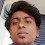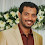# Basic Terms In Marine Electricity

What is Electricity?

> Electricity is the presence and flow of electric charge. Using electricity we can transfer energy in ways that allow us to do simple chores.

What is the Charge?

> Electric charge is a basic property of electrons, protons and other subatomic particles. > There are two types of electric charge: positive and negative (commonly carried by protons and electrons respectively)

What is Current?

> The flow of electron is known as current or in another word rate of flow of charge is known as current

>  It is written by I = dq/dt = q/t c/sec ampere (Amp)

What is the Direction of current?

> In an element the current direction is from higher potential to potential.

> The current flows from +ve to -ve whereas the electrons flow from -ve to +ve.

What is the Voltage or potential difference?

> Voltage is the energy required to move a unit charge through an element.

> It is denoted by ‘v’.

> Its unit is volt. 1 volt = 1 joule/coulomb = 1 newton meter/coulomb

> It is also known as absolute potential at this point.

What is Power?

> Power is the time rate of expanding or absorbing energy

> Unit of power is watts-W

> P=VI

What is the Potential drop or voltage drop?

> When current flows through an element the voltage produced across that element is known as voltage drop.

What is the Resistor?

> It is a device which opposes the flow of current.

What is the Resistance?

> It is the property of a resistor by virtue of which it opposes the flow of current. The mathematical expression of resistance is given by ohm’s law (see laws).

What is Resistivity?

> It is defined as the total resistance offered by a conductor per unit length.

What is the Inductor?

> It is a device which opposes any change inflow of current.

What is Inductance?

> It is the property of an inductor by which it opposes any change in the inflow of current.

> Unit of inductance is henry (H).

> It is represented by L.

> Mathematically it is given by L = NФ/I

Where

N = number of turns of the coil

Ф = Flux of the coil

I = current in the coil

What is the Inductive Reactance?

> It is the property of an inductor by which it opposes the flow of current.

It is given by; XL = 2πfL = wL

Where

f = natural frequency

w = angular frequency

What is a capacitor?

> The capacitor is a component which has the ability or “capacity” to store energy in the form of an electrical charge producing a potential difference (Static Voltage) across its plates, much like a small rechargeable battery.

What is capacitance?

> Capacitance is the electrical property of a capacitor and is the measure of a capacitors ability to store an electrical charge onto its two plates

> Unit of capacitor Farad (F)

1.1.